# Physics 10 - Work and Power.pptx

Jun. 2, 2023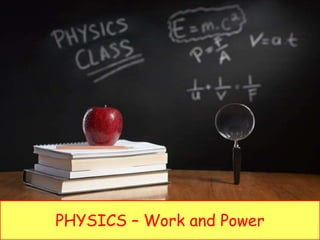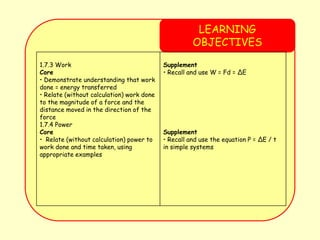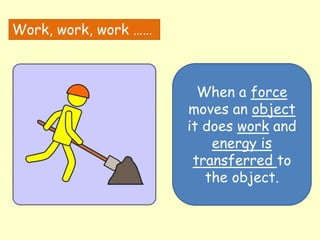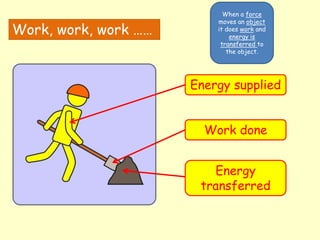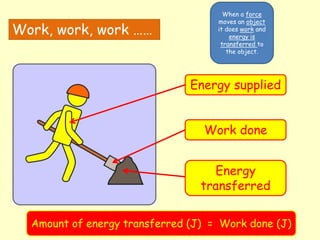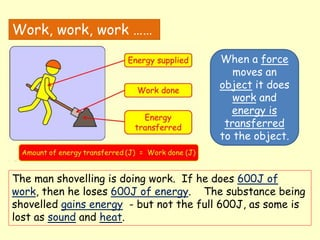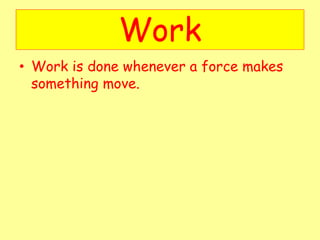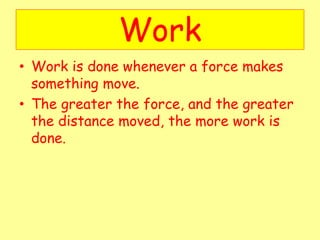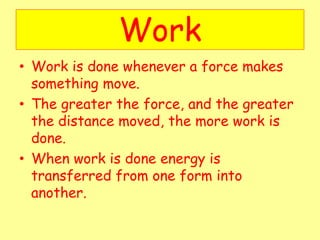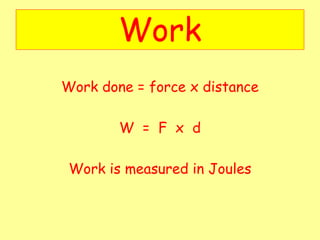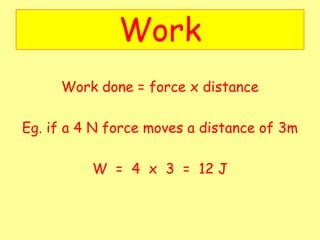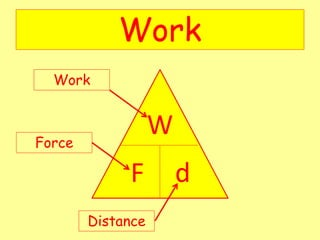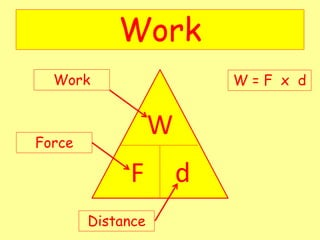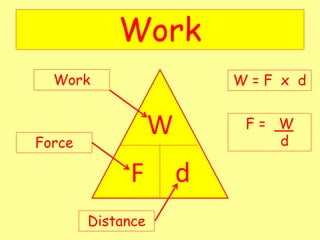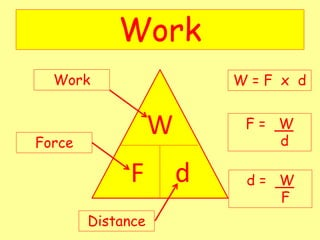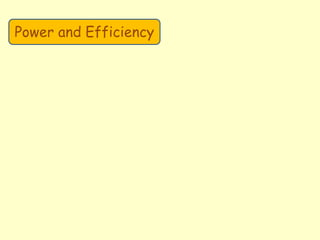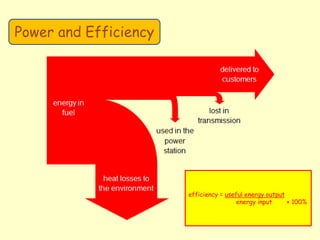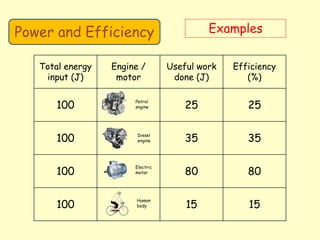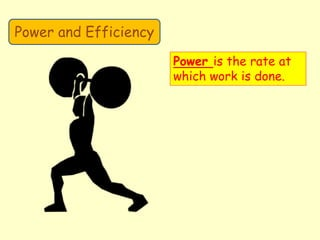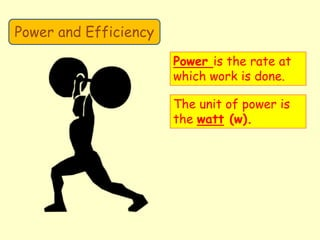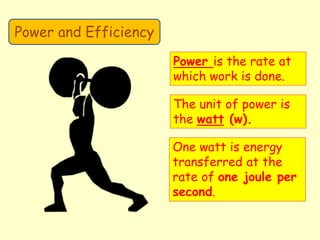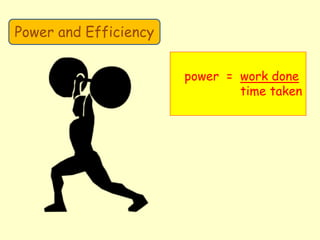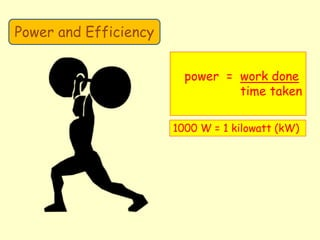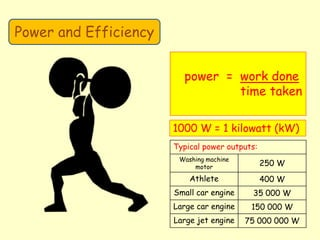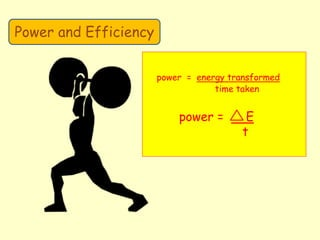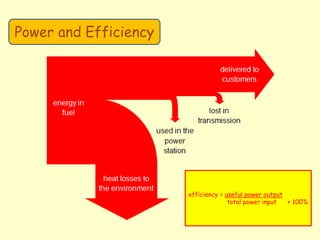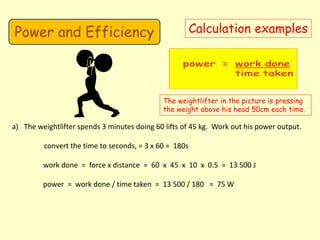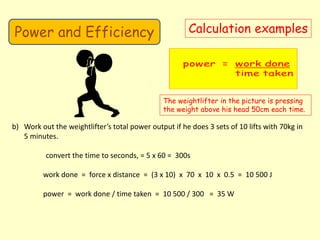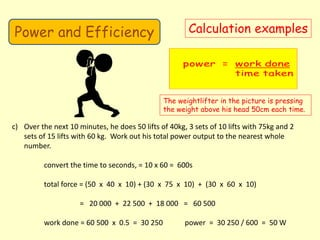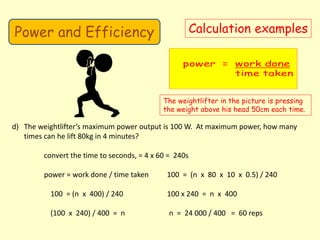1 of 34

### Physics 10 - Work and Power.pptx

• 1. PHYSICS – Work and Power
• 2. LEARNING OBJECTIVES 1.7.3 Work Core • Demonstrate understanding that work done = energy transferred • Relate (without calculation) work done to the magnitude of a force and the distance moved in the direction of the force 1.7.4 Power Core • Relate (without calculation) power to work done and time taken, using appropriate examples Supplement • Recall and use W = Fd = ∆E Supplement • Recall and use the equation P = ∆E / t in simple systems
• 4. Work, work, work …… When a force moves an object it does work and energy is transferred to the object.
• 5. Work, work, work …… When a force moves an object it does work and energy is transferred to the object. Energy supplied Work done Energy transferred
• 6. Work, work, work …… When a force moves an object it does work and energy is transferred to the object. Energy supplied Work done Energy transferred Amount of energy transferred (J) = Work done (J)
• 7. Work, work, work …… When a force moves an object it does work and energy is transferred to the object. The man shovelling is doing work. If he does 600J of work, then he loses 600J of energy. The substance being shovelled gains energy - but not the full 600J, as some is lost as sound and heat.
• 8. Work • Work is done whenever a force makes something move.
• 9. Work • Work is done whenever a force makes something move. • The greater the force, and the greater the distance moved, the more work is done.
• 10. Work • Work is done whenever a force makes something move. • The greater the force, and the greater the distance moved, the more work is done. • When work is done energy is transferred from one form into another.
• 11. Work Work done = force x distance W = F x d Work is measured in Joules
• 12. Work Work done = force x distance Eg. if a 4 N force moves a distance of 3m W = 4 x 3 = 12 J
• 18. efficiency = useful energy output energy input × 100% Power and Efficiency
• 19. Power and Efficiency Examples Total energy input (J) Engine / motor Useful work done (J) Efficiency (%) 100 25 25 100 35 35 100 80 80 100 15 15 Petrol engine Diesel engine Electric motor Human body
• 20. Power and Efficiency Power is the rate at which work is done.
• 21. Power and Efficiency Power is the rate at which work is done. The unit of power is the watt (w).
• 22. Power and Efficiency Power is the rate at which work is done. The unit of power is the watt (w). One watt is energy transferred at the rate of one joule per second.
• 23. Power and Efficiency power = work done time taken
• 24. Power and Efficiency power = work done time taken 1000 W = 1 kilowatt (kW)
• 25. Power and Efficiency power = work done time taken 1000 W = 1 kilowatt (kW) Typical power outputs: Washing machine motor 250 W Athlete 400 W Small car engine 35 000 W Large car engine 150 000 W Large jet engine 75 000 000 W
• 26. Power and Efficiency power = energy transformed time taken power = E t
• 27. efficiency = useful power output total power input × 100% Power and Efficiency
• 28. Power and Efficiency Calculation examples The weightlifter in the picture is pressing the weight above his head 50cm each time. a) The weightlifter spends 3 minutes doing 60 lifts of 45 kg. Work out his power output. convert the time to seconds, = 3 x 60 = 180s work done = force x distance = 60 x 45 x 10 x 0.5 = 13 500 J power = work done / time taken = 13 500 / 180 = 75 W
• 29. Power and Efficiency Calculation examples The weightlifter in the picture is pressing the weight above his head 50cm each time. b) Work out the weightlifter’s total power output if he does 3 sets of 10 lifts with 70kg in 5 minutes. convert the time to seconds, = 5 x 60 = 300s work done = force x distance = (3 x 10) x 70 x 10 x 0.5 = 10 500 J power = work done / time taken = 10 500 / 300 = 35 W
• 30. Power and Efficiency Calculation examples The weightlifter in the picture is pressing the weight above his head 50cm each time. c) Over the next 10 minutes, he does 50 lifts of 40kg, 3 sets of 10 lifts with 75kg and 2 sets of 15 lifts with 60 kg. Work out his total power output to the nearest whole number. convert the time to seconds, = 10 x 60 = 600s total force = (50 x 40 x 10) + (30 x 75 x 10) + (30 x 60 x 10) = 20 000 + 22 500 + 18 000 = 60 500 work done = 60 500 x 0.5 = 30 250 power = 30 250 / 600 = 50 W
• 31. Power and Efficiency Calculation examples The weightlifter in the picture is pressing the weight above his head 50cm each time. d) The weightlifter’s maximum power output is 100 W. At maximum power, how many times can he lift 80kg in 4 minutes? convert the time to seconds, = 4 x 60 = 240s power = work done / time taken 100 = (n x 80 x 10 x 0.5) / 240 100 = (n x 400) / 240 100 x 240 = n x 400 (100 x 240) / 400 = n n = 24 000 / 400 = 60 reps
• 32. LEARNING OBJECTIVES 1.7.3 Work Core • Demonstrate understanding that work done = energy transferred • Relate (without calculation) work done to the magnitude of a force and the distance moved in the direction of the force 1.7.4 Power Core • Relate (without calculation) power to work done and time taken, using appropriate examples Supplement • Recall and use W = Fd = ∆E Supplement • Recall and use the equation P = ∆E / t in simple systems
• 33. PHYSICS – Work and Power